# Specialized Deep Learning Architectures for Time Series Forecasting

Contents

Statistical algorithms have long been widely used for making forecasts with time series data. These classical algorithms, like Exponential Smoothing, and ARIMA models, prescribe the data generation process and require manual selections to account for factors like the trend, seasonality, and auto-correlation. However, modern data applications often deal with hundreds or millions of related time series. For example, a demand forecasting algorithm at Amazon may have to consider sales data from millions of products, and an engagement forecasting algorithm at Instagram may have to model metrics from millions of posts. Traditional forecasting methods learn characteristics of individual time series, and hence do not scale well because they fit a model for each time series and do not share parameters among them.

Deep learning provides a data-driven approach that makes a minimal set of assumptions to learn from multiple related time series. In the previous article, I did a detailed literature review on the state of statistical vs machine learning vs deep learning approaches for time series forecasting1. It is important to note that deep learning methods are not necessarily free of inductive biases. While DL models make very few assumptions about the features, inductive biases creep into the modeling process in the form of the architectural design of the DL model. This is why we see certain models perform better than others on certain tasks. For example, Convolutional Neural Networks work well with images due to their spatial inductive biases and translational equivariance. Hence a careful design of the DL model based on the application domain is critical. Over the past few years, several new DL models have been developed for forecasting applications. In this article, we will go over some of the most popular DL models, understand their inductive biases, implement them in PyTorch and compare their results on a dataset with multiple time series.

Before going any further, let’s look at some fundamental concepts that will help develop a better understanding of the models.

We will focus on the following two types of forecasting applications:

• Point forecasting: Our goal is to predict a single value, which is often the conditional mean or median of future observations.
• Probabilistic forecasting: Our goal here will be to predict the full distribution. Probabilistic forecasting captures the uncertainty of the future and hence plays a key role in automating and optimizing operational business processes. For example: in retail, it helps to optimize procurement, and inventory planning; in logistics, it enables efficient labor resource planning, delivery vehicle deployments, etc.

We further divide probabilistic forecasting into two categories:

• Parametric probabilistic forecasting: We directly predict the parameters of the hypothetical target distribution, for example, we predict the mean and standard deviation under the gaussian distribution assumption. Maximum likelihood estimation is commonly applied to estimate the corresponding parameters in this setting.
• Non-Parametric probabilistic forecasting: We predict a set of values corresponding to each quantile point of interest. Such models are commonly trained to minimize quantile loss.

Along with the time series data, a lot of models also incorporate covariates. Covariates provide additional information about an item being modeled or the time point corresponding to the item’s observed values. For example, covariates ensure that the information about the absolute or relative time is available to the model when we use the windowing approach during training.

• Item-dependent covariates, can be product id, product brand, category, product image, product text, etc. One common way to incorporate them into the modeling process is by using embeddings. Some numeric covariates can also be repeated along the time dimensions to be used together with the time series input.
• Time-depended covariates, can be product price, weekend, holidays, day-of-the-week, etc, or binary features like price adjustment, censoring, etc. This information can usually be modeled using the corresponding numeric values.

Covariates can also be both time and item dependent, for example, product-specific promotions.

DeepAR is an RNN-based probabilistic forecasting model proposed by Amazon that trains on a large number of related time series 2. Even though it trains on all time series, it is still a univariate model, i.e. it predicts a univariate target. It learns seasonal behavior and dependencies without any manual feature engineering. It can also incorporate cold items with limited historical data. Assuming $t_{0}$ as the forecast creation time, i.e. the time step at which the forecast for the future horizon must be generated, our goal is to model the following conditional distribution: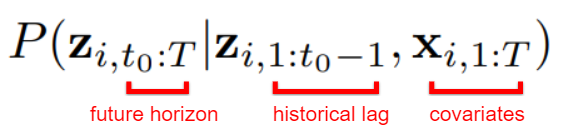Using the autoregressive recurrent network, we can further represent our model distribution as the product of likelihood factors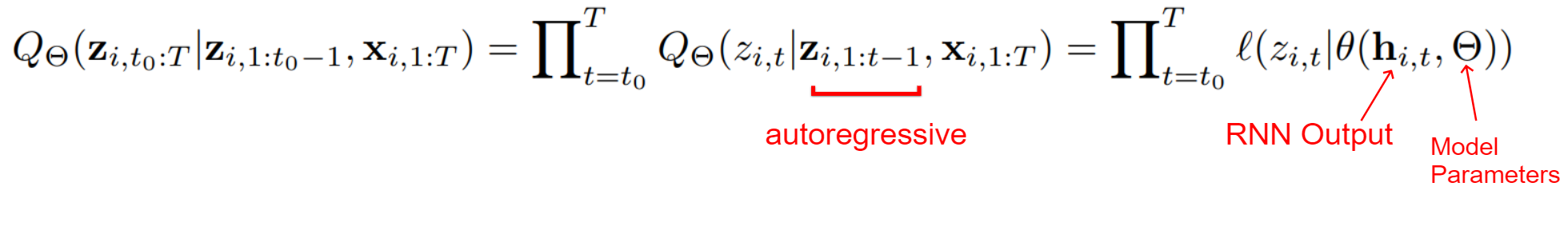parameterized by the output $h_{i,t}$ of an autoregressive recurrent network.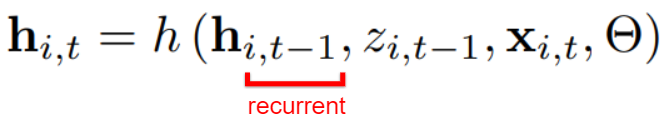Amazon’s website catalogs millions of products with very skewed sales velocity. The magnitudes of sales numbers among series also have a large variance which makes common normalization techniques like input standardization or batch normalization less effective. Also, the erratic, intermittent and bursty nature of data in such demand forecasting violates the core assumptions of many classical techniques, such as gaussian errors, stationarity, or homoscedasticity of the time series. The authors propose two solutions:

1. Scaling input time series: An average-based heuristic is used to scale the inputs. The autoregressive inputs $z_{i,t}$ are divided by the following average value $v_{i}$ and at the end, likelihood parameters are multiplied by the same value.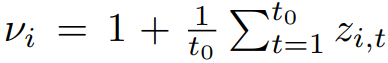2. Velocity-based sampling: Large magnitude variance can also lead to suboptimal results because an optimization procedure that picks training instances uniformly at random will visit the small number time series with a large scale very infrequently, which results in underfitting those time series. To handle this, a weighted sampling scheme is adopted where the probability of selecting a sample with scale $v_{i}$ is proportional to $v_{i}$ (see WeightedSampler class in code).

The paper also recommends using the following covariates: age (distance to the first observation in that time series), day-of-the-week and hour-of-the-week for hourly data, week-of-the-year for weekly data, month-of-the-year for monthly data, and a time series id (representing the product category) as embedding. All covariates were standardized to zero mean and unit variance.

DeepAR maximizes the log-likelihood but does not limit itself to assuming Gaussian noise. Any noise model can be chosen as long as the log-likelihood and its gradients wrt the parameters can be evaluated. The authors recommend using Gaussian distribution (parameterized by mean and standard deviation) for real-valued data, and negative-binomial likelihood (parameterized by mean and shape) for unbound positive count data and long-tail data. Other possibilities include Beta distribution for unit interval, Bernoulli for binary data, and mixtures for complex marginal distributions. The paper also recommends using the SoftPlus activation function for parameters, like standard deviation, that are always positive.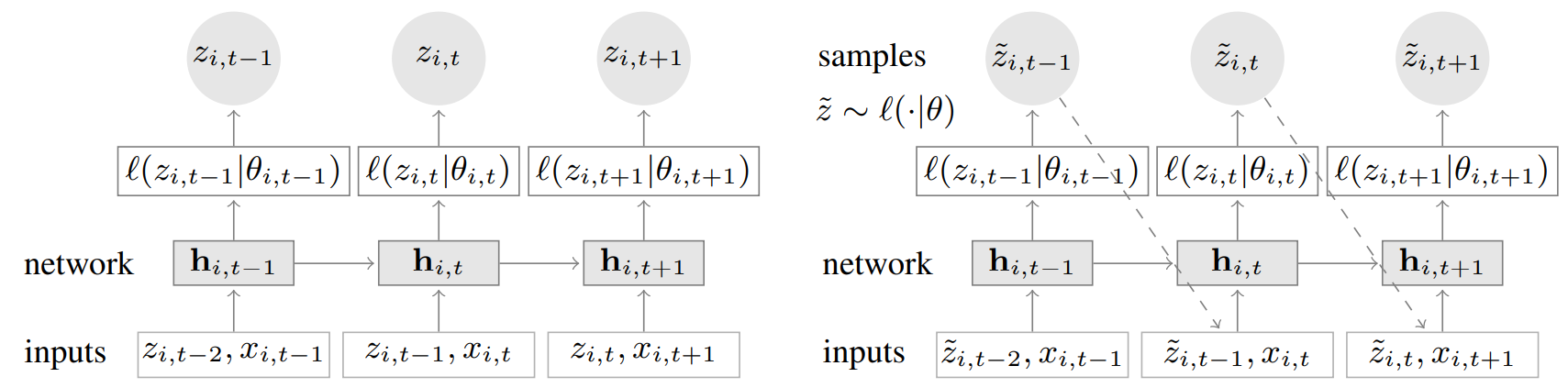DeepAR model uses an encoder-decoder design but uses the same architecture for the encoder and decoder components. During training (left), at each time step t, the model takes the target value at the previous time step $z_{i,t-1}$, covariates $x_{i,t}$, as well as the previous network output $h_{i,t-1}$. The model is using teacher forcing approach, which has been shown to pose a few problems for tasks like NLP but hasn’t had any known adverse effect in the forecasting setting. For prediction, the history of the time series is first fed for all timestamps before the forecast creation time (left), and a sample is drawn (also called ancestral sampling) and fed back for the next point until the end of the prediction range (right).

DeepAR model has been extended in several other research works. For example, DeepAR with quantiles functions 3 , DeepAR with dilated causal convolutions 4, and DeepAR for multivariate forecasting (TimeGrad) 5.

  1 2 3 4 5 6 7 8 9 10 11 12 13 14 15 16 17 18 19 20 21 22 23 24 25 26 27 28 29 30 31 32 33 34 35 36 37 38 39 40 41 42 43  class DeepAR(nn.Module): def __init__(self, num_class=num_series, embedding_dim=20, cov_dim=num_covariates, lstm_hidden_dim=40, lstm_layers=3, lstm_dropout=0.1, sample_times=200, predict_start=window_size-stride_size, predict_steps=stride_size, device=torch.device('cuda')): super(DeepAR, self).__init__() self.lstm_layers = lstm_layers self.lstm_hidden_dim = lstm_hidden_dim self.device = device self.sample_times = sample_times self.predict_steps = predict_steps self.predict_start = predict_start self.embedding = nn.Embedding(num_class, embedding_dim) self.lstm = nn.LSTM(input_size=1+cov_dim+embedding_dim, hidden_size=lstm_hidden_dim, num_layers=lstm_layers, bias=True, batch_first=False, dropout=lstm_dropout) self.relu = nn.ReLU() self.distribution_mu = nn.Linear(lstm_hidden_dim * lstm_layers, 1) self.distribution_presigma = nn.Linear(lstm_hidden_dim * lstm_layers, 1) self.distribution_sigma = nn.Softplus() def forward(self, x, idx, hidden, cell): onehot_embed = self.embedding(idx) lstm_input = torch.cat((x, onehot_embed), dim=2) output, (hidden, cell) = self.lstm(lstm_input, (hidden, cell)) hidden_permute = hidden.permute(1, 2, 0).contiguous().view(hidden.shape, -1) mu = self.distribution_mu(hidden_permute) pre_sigma = self.distribution_presigma(hidden_permute) sigma = self.distribution_sigma(pre_sigma) return torch.squeeze(mu), torch.squeeze(sigma), hidden, cell 

Refer to this Gist for the complete code for this experiment: https://gist.github.com/reachsumit/c1f7e11c0cfa5f696fd9ccd392f9b8d0

Multi-horizon Quantile Recurrent Neural Network (MQRNN) is an RNN-based non-parametric probabilistic forecasting model proposed by Amazon 6. It uses quantile loss to predict values for each desired quantile for every time step in the forecast horizon. One problem with recursive forecast generators like DeepAR, is that they tend to accumulate errors from previous steps during recursive forecast generation. Some empirical search also suggests that directly forecasting values for the full forecast horizon are less biased, more stable, and retrains the efficiency of the parameter sharing 7. MQRNN builds on this idea and uses direct multi-horizon forecasting instead of a one-step-ahead recursive forecasting approach. It also incorporates both static and temporal covariates and solves the following large-scale time series regression problem: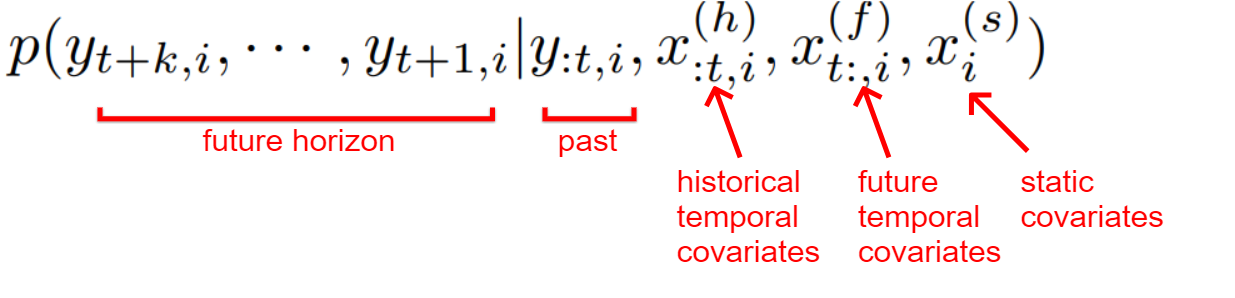This paper suggests that distant future information can have a retrospective impact on near-future horizons. For example, if a festival or black-Friday sales event is coming up in the future, the anticipation of it can affect a customer’s buying decisions. As explained later, this future information is supplied to the two decoder MLP components.

The model adopts the encoder-decoder framework. The encoder is a vanilla LSTM network that takes historical time series and covariates values. For the decoder structure, the model uses two MLP branches instead of a recursive decoder. As stated earlier, the model focuses on directly producing output for the full horizon at once. The MLP branches are used to achieve this goal. The design philosophy for the two decoders is as follows:

• Global Decoder: The global MLP takes encoder output and future covariates as input, and generates two context vectors as output: horizon-specific context, and horizon-agnostic context.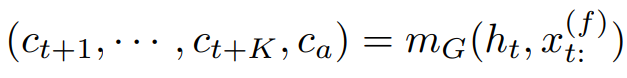The idea here behind horizon-specific context is that it carries the structural awareness of the temporal distance between a forecast creation time point and a specific horizon. This is essential to aspects like seasonality mapping. Whereas the horizon-agnostic context is based on the idea that not all relevant information is time-sensitive. Note that in the standard encoder-decoder architecture, only horizon-agnostic context exists, and horizon awareness is indirectly enforced by recursively feeding predictions not the RNN cell for the next time step.

• Local Decoder: The local MLP takes the global decoder’s outputs and also future covariates as input, and generates all required quantiles for each specific horizon.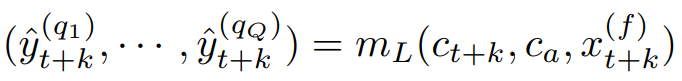The local MLP is key to aligning future seasonality and event, and the capability to generate sharp, spiky forecasts. In cases where there is no meaningful future information, or sharp, spiky forecasts are not desired, the local MLP can be removed.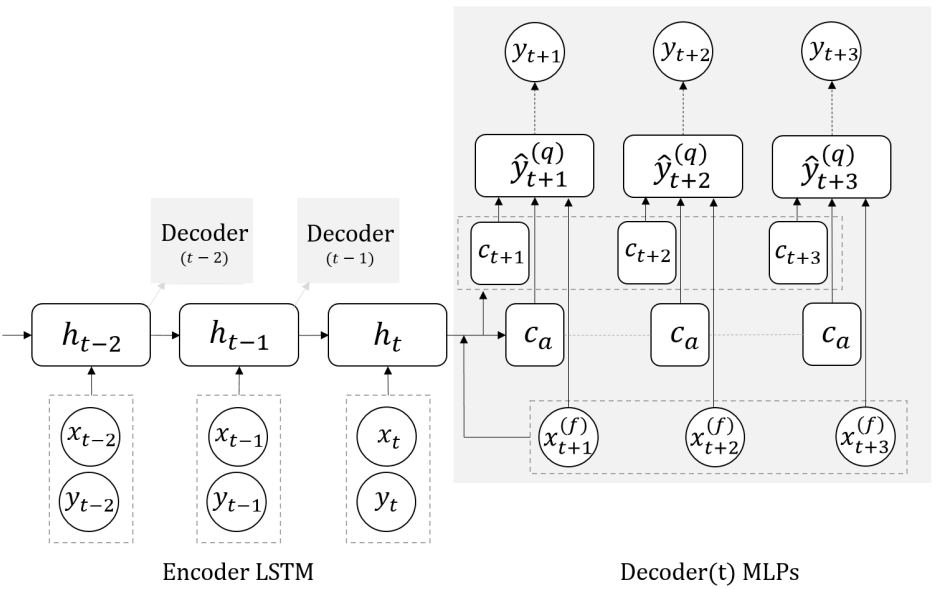The loss function for MQRNN is the sum of individual quantile loss, and the output is all the quantile forecasts. At 0.5 quantile, the quantile loss is simply the Mean Absolute Error and its minimizer is the median of the predictive distribution. MQRNN generates multi-horizon forecasts by placing a series of decoders, with shared parameters, at each recurrent layer (time point) in the encoder, and computes the loss against the corresponding targets. Each time series of arbitrary lengths can serve as a single sample in the model training, hence allowing cold content with limited history to also be used in the model. It also uses static information by replicating it across time. The authors also recommend trying different encoder structures for processing sequential input, like dilated 1D causal convolution layers (MQCNN), NARX-like LSTM, or WaveNet.

  1 2 3 4 5 6 7 8 9 10 11 12 13 14 15 16 17 18 19 20 21 22 23 24 25 26 27 28 29 30 31 32 33 34 35 36 37 38 39 40 41 42 43 44 45 46 47 48 49 50 51 52 53 54  class MQRNN(nn.Module): def __init__(self, horizon_size:int=horizon_size, hidden_size:int=100, quantiles:list=desired_quantiles, dropout:float=0.3, layer_size:int=3, context_size:int=50, covariate_size:int=num_covariates, bidirectional=False, device=torch.device('cuda')): super(MQRNN, self).__init__() self.quantiles = desired_quantiles self.quantile_size = len(quantiles) self.bidirectional = bidirectional self.hidden_size = hidden_size self.horizon_size = horizon_size self.device = device self.covariate_size = covariate_size self.encoder = nn.LSTM(input_size= covariate_size+1, hidden_size=hidden_size, num_layers=layer_size, dropout=dropout, bidirectional=bidirectional) self.global_decoder = nn.Sequential(nn.Linear(in_features= hidden_size + covariate_size*horizon_size, out_features= horizon_size*hidden_size*3), nn.ReLU(), nn.Linear(in_features= horizon_size*hidden_size*3, out_features= horizon_size*hidden_size*2), nn.ReLU(), nn.Linear(in_features= horizon_size*hidden_size*2, out_features= (horizon_size+1)*context_size), nn.ReLU()) self.local_decoder = nn.Sequential(nn.Linear(in_features= horizon_size*context_size + horizon_size* covariate_size + context_size, out_features= horizon_size* context_size), nn.ReLU(), nn.Linear(in_features= horizon_size* context_size, out_features= horizon_size*self.quantile_size), nn.ReLU()) self.encoder.double().to(self.device) self.global_decoder.double().to(self.device) self.local_decoder.double().to(self.device) def forward(self, cur_series_covariate_tensor, next_covariate_tensor): seq_len, batch_size = cur_series_covariate_tensor.shape, cur_series_covariate_tensor.shape direction_size = 2 if self.bidirectional else 1 outputs,_ = self.encoder(cur_series_covariate_tensor) outputs_reshape = outputs.view(seq_len,batch_size,direction_size,self.hidden_size)[:,:,-1,:] encoder_outputs = outputs_reshape.view(seq_len,batch_size,self.hidden_size) if not self.training: encoder_outputs = torch.unsqueeze(encoder_outputs[-1],dim=0) global_decoder_output = self.global_decoder(torch.cat([encoder_outputs, next_covariate_tensor], dim=2)) local_decoder_output = self.local_decoder(torch.cat([global_decoder_output, next_covariate_tensor], dim=2)) seq_len = local_decoder_output.shape batch_size = local_decoder_output.shape return local_decoder_output.view(seq_len, batch_size, self.horizon_size, self.quantile_size) 

Refer to this Gist for the complete code for this experiment: https://gist.github.com/reachsumit/b0dfc7390fe4eff023226bbb6c0f538b

You can read more about MQRNN in the original paper and the official codebase.

Another clever usage of the encoder-decoder framework for time-series forecasting was proposed by Uber 8. The main objective of this proposal was to create a solution for quantifying the forecasting uncertainty using Bayesian Neural Networks. This approach can also be used for anomaly detection at scale. The bayesian perspective introduces uncertainty measurement to deep learning models. A prior (often Gaussian) is introduced for the weight parameters, and the model aims to fit the optimal posterior distribution. Many methods, such as stochastic search, variational Bayes, and probabilistic backpropagation, have been proposed to approximate inference methods for Bayesian networks at scale. This paper adopts a Monte Carlo Dropout based approach suggested by prior research 9. Specifically, stochastic dropouts are applied after each hidden layer, and the model output can be approximately viewed as a random sample generated from posterior prediction distribution. Hence the uncertainty in model predictions can be estimated by the sample variance of the model predictions in a few repetitions.

This paper decomposes prediction uncertainty into 3 types:

• Uncertainty due to model (ignorance of model parameters). This uncertainty can be reduced by collecting more samples or by using MC dropouts.
• Uncertainty due to data (train and test samples are from a different population). This can be accounted for by fitting a latent embedding space for all training time series using an encoder-decoder model and calculating variance using only the encoder representations.
• Inherent noise (irreducible). This noise can be estimated through the residual sum of squares evaluated on an independent help-out validation set.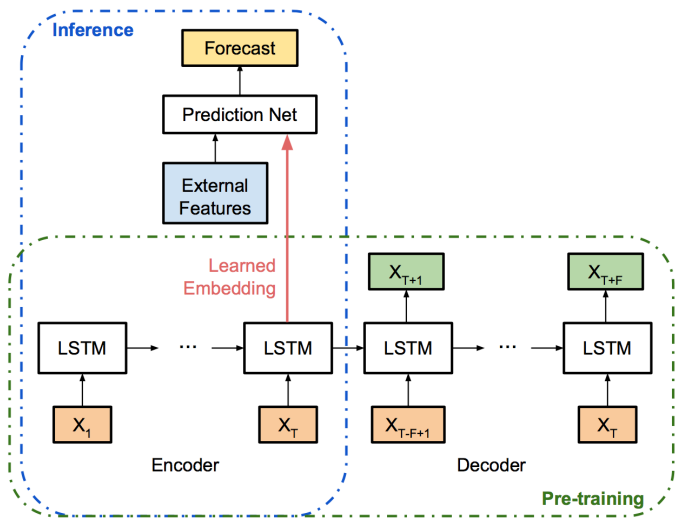The encoder-decoder is a standard RNN-based framework that captures the inherent pattern in the time series during the pre-training step. After the encoder-decoder is pre-trained, the encoder is treated as an intelligent feature-extraction blackbox. A prediction network learns to take input from both the learned encoder, as well as any potential external features to generate the final prediction. Before training, the raw data are log-transformed to remove exponential effects. Within each input sliding window, the first day is subtracted from all values, so that trends are removed and the neural network is trained for the increment value. Later, these transformations are reverted to obtain predictions at the original scale. In the paper, learned embeddings (i.e. the last LSTM cell states in the encoder) are projected to lower dimensions using PCA for interpreting extracted features.

  1 2 3 4 5 6 7 8 9 10 11 12 13 14 15 16 17 18 19 20 21 22 23 24 25 26 27 28 29 30 31 32 33 34 35 36 37 38 39 40 41 42 43 44 45 46 47 48 49 50 51 52 53 54 55 56 57 58 59 60 61 62 63 64 65 66 67 68 69 70 71 72 73 74 75 76 77 78 79 80 81 82 83  class VariationalDropout(nn.Module): def __init__(self, dropout): super().__init__() self.dropout = dropout def forward(self, x: torch.Tensor) -> torch.Tensor: if not self.training: return x max_batch_size = x.size(1) m = x.new_empty(1, max_batch_size, x.size(2), requires_grad=False).bernoulli_(1 - self.dropout) x = x.masked_fill(m == 0, 0) / (1 - self.dropout) return x class LSTM(nn.LSTM): def __init__(self, *args, dropouti=0., dropoutw=0., dropouto=0., unit_forget_bias=True, **kwargs): super().__init__(*args, **kwargs) self.unit_forget_bias = unit_forget_bias self.dropoutw = dropoutw self.input_drop = VariationalDropout(dropouti) self.output_drop = VariationalDropout(dropouto) def forward(self, input): input = self.input_drop(input) seq, state = super().forward(input) return self.output_drop(seq), state class VDEncoder(nn.Module): def __init__(self, in_features, out_features, p): super(VDEncoder, self).__init__() self.model = nn.ModuleDict({ 'lstm1': LSTM(in_features, 32,dropouto=p), 'lstm2': LSTM(32, 8, dropouto=p), 'lstm3': LSTM(8, out_features, dropouto=p) }) def forward(self, x): out, _ = self.model['lstm1'](x) out, _ = self.model['lstm2'](out) out, _ = self.model['lstm3'](out) return out class VDDecoder(nn.Module): def __init__(self, p): super(VDDecoder, self).__init__() self.model = nn.ModuleDict({ 'lstm1': LSTM(1, 2, dropouto=p), 'lstm2': LSTM(2, 2, dropouto=p), 'lstm3': LSTM(2, 1, dropouto=p) }) def forward(self, x): out, _ = self.model['lstm1'](x) out, _ = self.model['lstm2'](out) out, _ = self.model['lstm3'](out) return out class VDEncoderDecoder(nn.Module): def __init__(self, in_features, output_steps, p): super(VDEncoderDecoder, self).__init__() self.enc_in_features = in_features self.output_steps = output_steps self.enc_out_features = 1 self.model = nn.ModuleDict({ 'encoder': VDEncoder(self.enc_in_features, self.enc_out_features, p), 'decoder': VDDecoder(p), 'fc1': nn.Linear(window_size, 32), 'fc2': nn.Linear(32, self.output_steps) }) def forward(self, x): out = self.model['encoder'](x) out = self.model['decoder'](out) out = self.model['fc1'](out.squeeze().view(64,-1)) out = self.model['fc2'](out) return out 

Refer to this Gist for the complete code for this experiment: https://gist.github.com/reachsumit/f4a55186706675a085157c64fd1e0634

The Deep Temporal Convolutional Network (DeepTCN) was proposed by Bigo Research 10. Instead of using RNNs, DeepTCN utilizes stacked layers of dilated causal convolutional nets to capture the long-term correlations in time series data. RNNs can be remarkably difficult to train due to exploding or vanishing gradients 11, and backpropagation through time (BPTT) often hampers efficient computation 12. On the other hand, Temporal Convolutional Network (TCN) is more robust to error accumulation due to its non-autoregressive nature, and it can also be efficiently trained in parallel.

A TCN is a 1D FCN with causal convolutions. Casual means that there is no information leakage from future to past, i.e. the output at time t can only be obtained from the inputs that are no later than t. Dilated convolutions enable exponentially large receptive fields (as opposed to linear to the depth of the network). Dilation allows the filter to be applied over an area larger than its length by skipping the input values by a certain step.

Recent studies have empirically shown that TCNs outperform RNNs across a broad range of sequence modeling tasks. These studies also recommend using TCNs, instead of RNNs, as the default starting point for sequence modeling tasks 13.

This research used time-independent covariates like product_id to incorporate series-level information that helps in capturing the scale level and seasonality of each specific series. Other covariates included hour-of-the-day, day-of-the-week, and day-of-the-month for hourly data, day-of-the-year for daily data, and month-of-the-year for monthly data. One of the major concerns for this study was to effectively handle complex seasonal patterns, like holiday effects. This is done by using a hand-crafted exogenous variable (covariates) such as holiday indicators, weather forecasts, etc. New products with little historical information used zero-padding to ensure the desired input sequence length.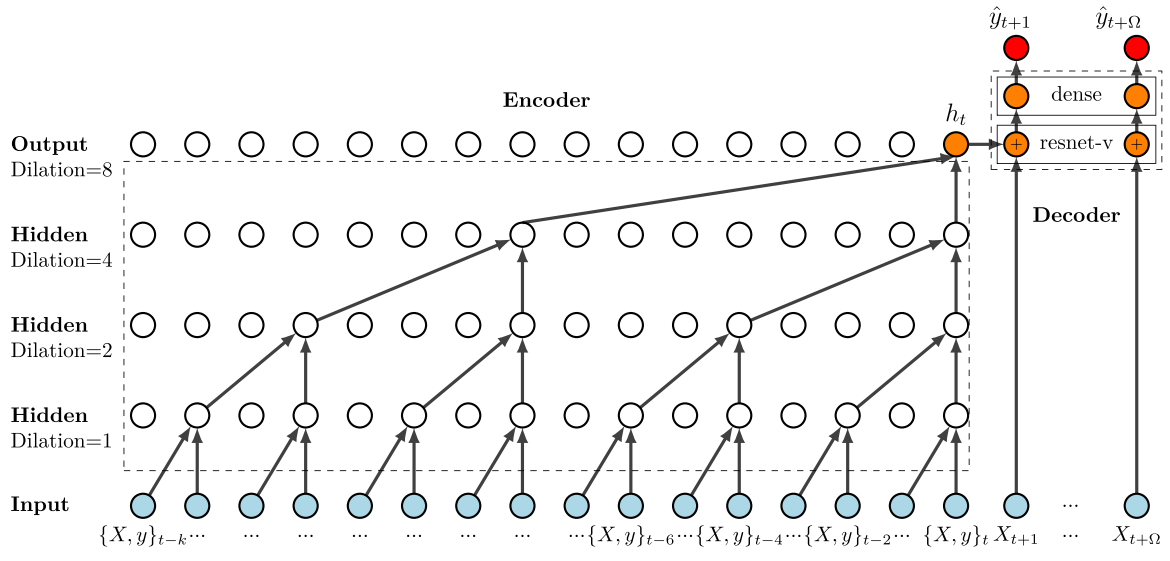DeepTCN uses an encoder-decoder framework. The encoder is composed of stacked residual blocks based on dilated causal convolutional nets to capture temporal dependencies. The diagram above shows an encoder on the left with {1, 2, 4, 8} as the dilation factors, filter size of 2, and receptive field of 16. More specifically, the encoder architecture is stacked dilated causal convolutions that model historical observations and covariates. The diagram below shows the original DeepTCN encoder on the left, and the one I adapted from another research13 for my implementation.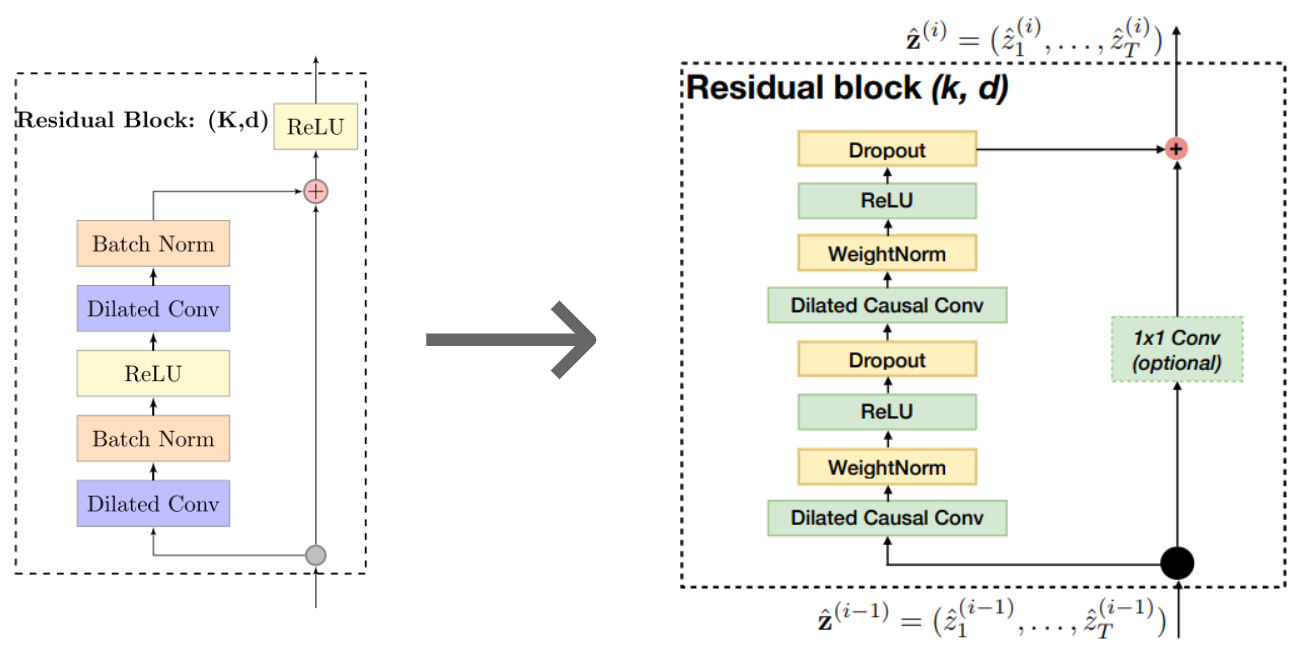For the decoder, the paper proposed a modified resnet-v block that takes two inputs (encoder output and future covariates). The decoder architecture is shown in the figure below.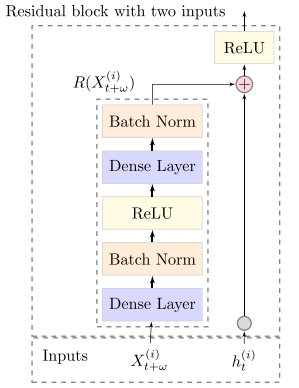1 2 3 4 5 6 7 8 9 10 11 12 13 14 15 16 17 18 19 20 21 22 23 24 25 26 27 28 29 30 31 32 33 34 35 36 37 38 39 40  class ResidualBlock(nn.Module): def __init__(self, input_dim, d, stride=1, num_filters=35, p=0.2, k=2, weight_norm=True): super(ResidualBlock, self).__init__() self.k, self.d, self.dropout_fn = k, d, nn.Dropout(p) self.conv1 = nn.Conv1d(input_dim, num_filters, kernel_size=k, dilation=d) self.conv2 = nn.Conv1d(num_filters, num_filters, kernel_size=k, dilation=d) if weight_norm: self.conv1, self.conv2 = nn.utils.weight_norm(self.conv1), nn.utils.weight_norm(self.conv2) self.downsample = nn.Conv1d(input_dim, num_filters, 1) if input_dim != num_filters else None def forward(self, x): out = self.dropout_fn(F.relu(self.conv1(x.float()))) out = self.dropout_fn(F.relu(self.conv2(out))) residual = x if self.downsample is None else self.downsample(x) return F.relu(out + residual[:,:,-out.shape:]) class FutureResidual(nn.Module): def __init__(self, in_features): super(FutureResidual, self).__init__() self.net = nn.Sequential(nn.Linear(in_features=in_features, out_features=in_features), nn.BatchNorm1d(in_features), nn.ReLU(), nn.Linear(in_features=in_features, out_features=in_features), nn.BatchNorm1d(in_features),) def forward(self, lag_x, x): out = self.net(x.squeeze()) return F.relu(torch.cat((lag_x, out), dim=2)) class DeepTCN(nn.Module): def __init__(self, cov_dims=cov_dims, num_class=num_series, embedding_dim=20, dilations=[1,2,4,8,16,24,32], p=0.25, device=torch.device('cuda')): super(DeepTCN, self).__init__() self.input_dim, self.cov_dims, self.embeddings, self.device = 1+(len(cov_dims)*embedding_dim), cov_dims, [], device for cov in cov_dims: self.embeddings.append(nn.Embedding(num_class, embedding_dim, device=device)) self.encoder = nn.ModuleList() for d in dilations: self.encoder.append(ResidualBlock(input_dim=self.input_dim, num_filters=self.input_dim, d=d)) self.decoder = FutureResidual(in_features=self.input_dim-1) self.mlp = nn.Sequential(nn.Linear(1158, 8), nn.BatchNorm1d(8), nn.SiLU(), nn.Dropout(p), nn.Linear(8,1), nn.ReLU()) 

Refer to this Gist for the complete code for this experiment: https://gist.github.com/reachsumit/e0a56592c32844231d40e3e48a1bc64a

N-BEATS is a univariate time series forecasting model proposed by Element AI (acquired by ServiceNow) 14. This research work came out at the time when, ES-RNN, a hybrid of Exponential Smoothing and RNN model won the M4 competition in 2018. A popular narrative at that time suggested that the hybrid of statistical and deep learning methods could be the way forward. But this paper challenged this notion by developing a pure-DL architecture for time series forecasting that was inspired by the signal processing domain. Their architecture also allowed for interpretable outputs by carefully injecting a suitable inductive bias into the model. However, there is no provision to include covariates in the model. N-BEATS was later extended by N-BEATSx 15 to incorporate exogenous variables. Another work, N-HiTS 16 altered the architecture and achieved accuracy improvements along with drastically cutting long-forecasting compute costs.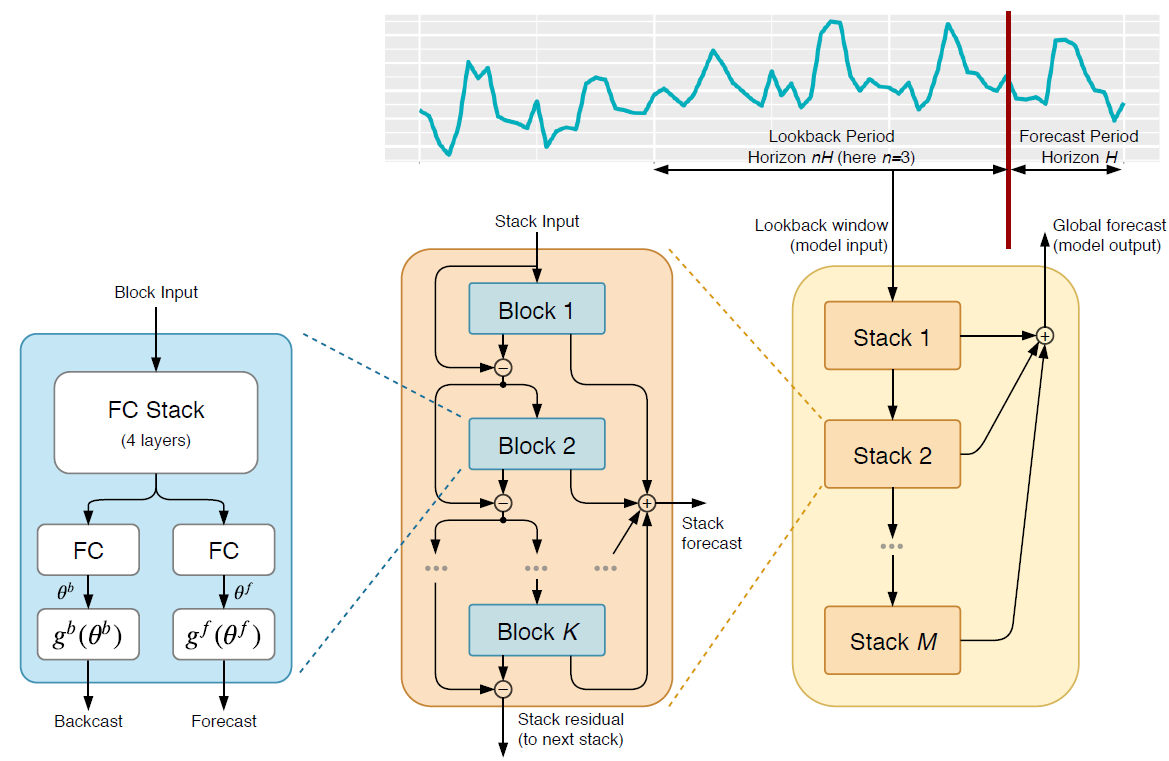The above diagram shows the N-BEATS architecture from the most granular view on the left to the high-level view on the right. N-BEATS used the residual principle to stack many basic blocks and the paper has shown that we can stack up to 150 layers and still facilitate efficient learning. Let’s look at each of the above 3 columns individually.

• [Left] Block: Each block takes the lookback period data as input and generates two outputs: a backcast and a forecast. The backcast is the block’s own best prediction of the lookback period. The input to the block is first processed by four standard fully connected layers (with bias and activation) and output from this FC stack is transformed by two separate linear layers (no bias or activation) to something the paper calls expansion coefficients for the backcast and forecast, $\theta^{b}$, and $\theta^{f}$, respectively. These expansion coefficients are then mapped to output using a set of basis layers ($g^{b}$ and $g^{f}$).
• [Middle] Stacks: Different blocks are arranged in a stack. All the blocks in a stack share the same kind of basis layer. The blocks are arranged in a residual manner, such that the input to a block $l$ is $x_{l} = x_{l-1} - \hat{x}_{l-1}$, i.e. at each step, the backcast generated by the block is subtracted from the input to that block before it is passed on to the next layer. And all the forecast outputs of all the blocks in a stack are added up to make the stack forecast. The residual backcast from the last block in a stack is the stack residual
• [Right] Overall Architecture: On the right, we see the top-level view of the architecture. Each stack is chained together so that for any stack, the stack residual output of the previous stack is the input and the stack generates two outputs: the stack forecast and the stack residual. Finally, the N-BEATS forecast is the additive sum of all the stack forecasts.

Basis Layer

A basis layer puts a constraint on the functional space and thereby limits the output representation constrained by the chosen basis function. We can have the basis of any arbitrary function, which gives us a lot of flexibility. In the paper, N-BEATS operates in two modes: generic and interpretable. The generic mode doesn’t have any basis function constraining the function search (we can think of it as having an identity function). So, in this mode, we are leaving the function completely learned by the model through a linear projection of the basis coefficients. Fixing the basis function allows for human interpretability about what each stack signifies. The authors propose two specific basis functions that capture trend (polynomial function) and seasonality (Fourier basis). So we can say that the forecast output of the stack represents trend or seasonality based on the corresponding chosen basis function.

  1 2 3 4 5 6 7 8 9 10 11 12 13 14 15 16 17 18 19 20 21 22 23 24 25 26 27 28 29 30 31 32 33 34 35 36 37 38 39 40 41 42 43 44  class GenericBasis(nn.Module): def __init__(self, backcast_size, forecast_size): super().__init__() self.backcast_size, self.forecast_size = backcast_size, forecast_size def forward(self, theta): return theta[:, :self.backcast_size], theta[:, -self.forecast_size:] class NBeatsBlock(nn.Module): def __init__(self, input_size, theta_size: int, basis_function: nn.Module, layers: int, layer_size: int): super().__init__() self.layers = nn.ModuleList([nn.Linear(in_features=input_size, out_features=layer_size)] + [nn.Linear(in_features=layer_size, out_features=layer_size) for _ in range(layers - 1)]) self.basis_parameters = nn.Linear(in_features=layer_size, out_features=theta_size) self.basis_function = basis_function def forward(self, x: torch.Tensor): block_input = x for layer in self.layers: block_input = torch.relu(layer(block_input)) basis_parameters = self.basis_parameters(block_input) return self.basis_function(basis_parameters) class NBeats(nn.Module): def __init__(self, blocks: nn.ModuleList): super().__init__() self.blocks = blocks def forward(self, x: torch.Tensor, input_mask: torch.Tensor) -> torch.Tensor: residuals = x.flip(dims=(1,)) input_mask = input_mask.flip(dims=(1,)) forecast = x[:, -1:] for i, block in enumerate(self.blocks): backcast, block_forecast = block(residuals) residuals = (residuals - backcast) * input_mask forecast = forecast + block_forecast return forecast 

Refer to this Gist for the complete code for this experiment: https://gist.github.com/reachsumit/9b31afe74c560c9f804081af3e1b4a1d

I used UCI’s ElectricityLoadDiagrams20112014 dataset17 to run a quick experiment with minimal PyTorch implementations of these models. The dataset contains 370 time series sampled at 15 mins with a total of 140K observations for each series. The data were resampled to 1 hour. Three covariates (weekday, hour, month) and one time series id was used wherever allowed by the model architecture. Refer to the notebooks linked in respective sections to see the code for each experiment. The following chart shows RMSE values for the test set for each of the models. Results are sorted from left to right by the best to the worst.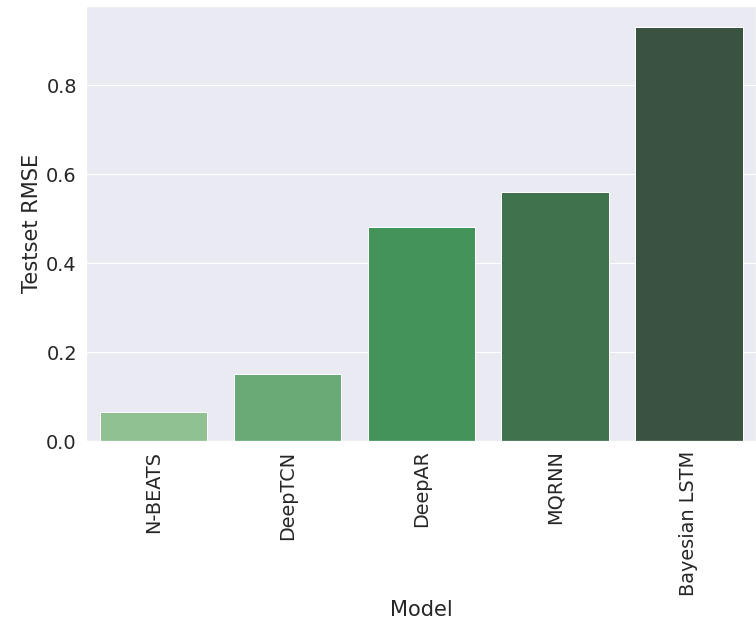In this article, we defined the need for using deep learning for modern time series forecasting and then looked at some of the most popular deep learning algorithms designed for time series forecasting with different inductive biases in their model architecture. We implemented all of the algorithms in Python and compared their results on a toy dataset.

1. “Statistical vs Machine Learning vs Deep Learning Modeling for Time Series Forecasting”, https://blog.reachsumit.com/posts/2022/12/stats-vs-ml-for-ts/ ↩︎

2. Flunkert, Valentin & Salinas, David & Gasthaus, Jan. (2017). DeepAR: Probabilistic Forecasting with Autoregressive Recurrent Networks. International Journal of Forecasting. 36. 10.1016/j.ijforecast.2019.07.001. ↩︎

3. Jan Gasthaus, Konstantinos Benidis, Yuyang Wang, Syama Sundar Rangapuram, David Salinas, Valentin Flunkert, and Tim Januschowski. Probabilistic forecasting with spline quantile function RNNs. In The 22nd International Conference on Artificial Intelligence and Statistics, pages 1901–1910, 2019. ↩︎

4. oord, Aaron & Dieleman, Sander & Zen, Heiga & Simonyan, Karen & Vinyals, Oriol & Graves, Alex & Kalchbrenner, Nal & Senior, Andrew & Kavukcuoglu, Koray. (2016). WaveNet: A Generative Model for Raw Audio. ↩︎

5. Kashif Rasul, Calvin Seward, Ingmar Schuster, and Roland Vollgraf. Autoregressive denoising diffusion models for multivariate probabilistic time series forecasting. In International Conference on Machine Learning, pages 8857–8868. PMLR, 2021. ↩︎

6. Wen, Ruofeng & Torkkola, Kari & Narayanaswamy, Balakrishnan. (2017). A Multi-Horizon Quantile Recurrent Forecaster. ↩︎

7. Ben Taieb, Souhaib & Atiya, Amir. (2015). A Bias and Variance Analysis for Multistep-Ahead Time Series Forecasting. IEEE transactions on neural networks and learning systems. 27. 10.1109/TNNLS.2015.2411629. ↩︎

8. Zhu, Lingxue & Laptev, Nikolay. (2017). Deep and Confident Prediction for Time Series at Uber. ↩︎

9. Gal, Yarin & Ghahramani, Zoubin. (2015). Dropout as a Bayesian Approximation: Appendix. ↩︎

10. Chen, Yitian & Kang, Yanfei & Chen, Yixiong & Wang, Zizhuo. (2019). Probabilistic Forecasting with Temporal Convolutional Neural Network. ↩︎

11. Pascanu, Razvan & Mikolov, Tomas & Bengio, Y.. (2012). On the difficulty of training Recurrent Neural Networks. 30th International Conference on Machine Learning, ICML 2013. ↩︎

12. Werbos, Paul. (1990). Backpropagation through time: what it does and how to do it. Proceedings of the IEEE. 78. 1550 - 1560. 10.1109/5.58337. ↩︎

13. Bai, Shaojie & Kolter, J. & Koltun, Vladlen. (2018). An Empirical Evaluation of Generic Convolutional and Recurrent Networks for Sequence Modeling. ↩︎ ↩︎

14. Oreshkin, Boris & Carpo, Dmitri & Chapados, Nicolas & Bengio, Yoshua. (2019). N-BEATS: Neural basis expansion analysis for interpretable time series forecasting. ↩︎

15. Gutierrez, Kin & Challu, Cristian & Marcjasz, Grzegorz & Weron, Rafał & Dubrawski, Artur. (2022). Neural basis expansion analysis with exogenous variables: Forecasting electricity prices with NBEATSx. International Journal of Forecasting. 10.1016/j.ijforecast.2022.03.001. ↩︎

16. Challu, Cristian & Gutierrez, Kin & Oreshkin, Boris & Garza, Federico & Mergenthaler, Max & Dubrawski, Artur. (2022). N-HiTS: Neural Hierarchical Interpolation for Time Series Forecasting. ↩︎